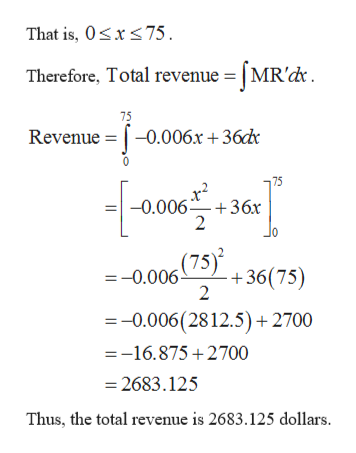# If the marginal revenue(in dollars per unit) for a month is given by MR'= -0.006x+36, find the total revenue from the sale of 75 units.

Question
112 views

If the marginal revenue(in dollars per unit) for a month is given by MR'= -0.006x+36, find the total revenue from the sale of 75 units.

check_circle

Step 1

The marginal revenue for a month is MR′ = -0.006x + 36.

The total r...help_outlineImage TranscriptioncloseThat is, 0sx75 Therefore, Total revenue = [MR'dk 75 Revenue -0.006x+36d 75 x -0.006 36x 2 =-0.006)36(75) 2 =-0.006(28 12.5) +2700 =-16.875+2700 =2683.125 Thus, the total revenue is 2683.125 dollars fullscreen

### Want to see the full answer?

See Solution

#### Want to see this answer and more?

Solutions are written by subject experts who are available 24/7. Questions are typically answered within 1 hour.*

See Solution
*Response times may vary by subject and question.
Tagged in
MathCalculus

### Other